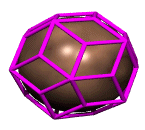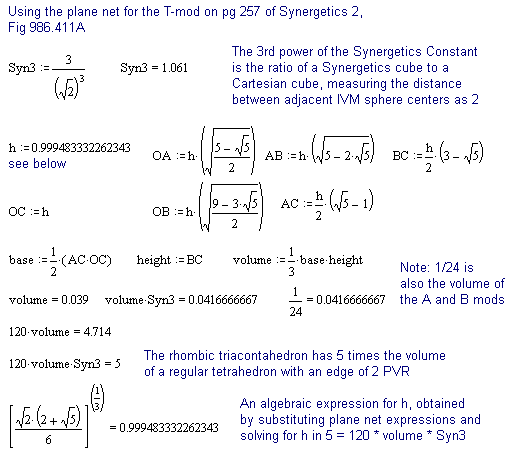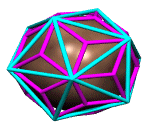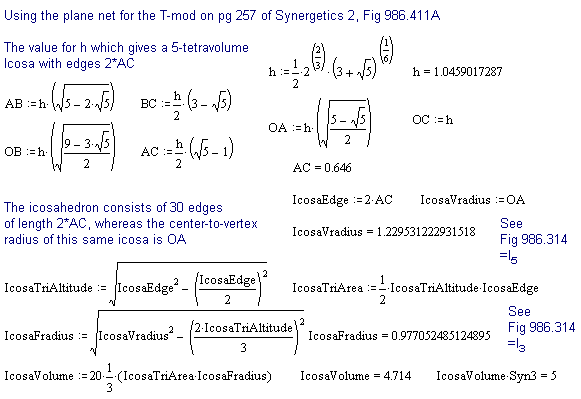The T-Module in Synergetics

The Rhombic Triacontahedron of 5-tetravolumes

The T-module is 120th of a rhombic triacontahedron -- a polyhedron consisting of 30 diamond-shaped (rhombic) faces. Each diamond has a long and short diagonal, which cross at the face center, thereby dividing the diamond into four triangles. The T-module consists of one such triangle and the three radial edges linking its three vertices to the center of the shape.

In Synergetics, the rhombic triacontahedron consisting of 120 T-modules weighs in at volume 5, meaning each of the 120 Ts has a volume of 1/24, equivalent to the volumes of the A and B modules. The rhombic triacontahedron with this volume has a body center to face center radius of length h, which is also the altitude of the T-module. It turns out that h is just shy of being the Prime Vector Radius (the radius of an IVM sphere).The calculations below show one way to calculate the volume of the rhombic triacontahedron, using the usual 1/3 x base x height method, multiplying by 120, and then multiplying the result by Syn3, the third power of the Synergetics Constant. This last step is required to adjust for the fact that Synergetics uses a different model of third powering, i.e. sets the tetrahedron defined by 4 unit-radius spheres packed together as volumetric unity.The Icosahedron of 5-tetravolumes

The rhombic triacontahedron's 30 long diagonals define an icosahedron, while its 30 short diagonals define a regular (pentagonal) dodecahedron. So using the same plane net for the T-module as above, we can adjust the value of h to get a differently sized rhombic triacontahedron (and corresponding long-diagonal icosahedron), this time with an eye towards making the icosahedron's volume come out to 5, versus the rhombic triacontahedron's.

The calculations below (which use the same T-mod plane net as above) are performed as a check on the derived value for h that will produce the desired result. The values obtained for the resulting icosa's body center to vertex and body center to mid face dimensions provide a check for Fig. 986.314 in Synergetics 2 (and a few more digits of accuracy), which was originally developed by Fuller's associates Robert Grip and Chris Kitrick. Again, the Syn3 is used to convert the resulting volume into synergetics' tetravolumes.Ongoing Explorations

Since Fuller's time, further work on the T-module has been undertaken by David Koski.

Koski has shown that:

• the T-mod is self-recursively assembled from phi-scaled T-modules
• Phi-scaled T-mods face-bond to assemble the so-called fat and thin rhombohedra and thereby certain 5-fold symmetrical shapes
• Phi-scaled T-mods, plus equivolumed "alternates" all carved from the golden cuboid, assemble many additional five-fold shapes
When it comes to computing the volumes of generic tetrahedra, we have another method based simply on providing the six edge lengths. Leonhard Euler developed one such algebraic expression. Gerald de Jong derived one as well, independently of Euler, and modified it to output tetravolumes directly -- in the form of a Java applet (which includes an animation relating the algebra to the geometry of the tetrahedron).

Note: Gerald's applet values the distance between adjacent IVM sphere centers as one, so, for example, you would need to divide the above plane net values in half in order to get volume = 5 in each instance.

Synergetics on the Web
maintained by Kirby Urner